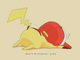# Parametric Graphing

### Overview

Try it out by typing a parametric equation in the form (sin(t), cos(t)). Here's an example: https://www.desmos.com/calculator/ksjcpazwa911 out of 15 found this helpful
Have more questions? Submit a request

•Hiroshmendis

thank for the help

•Fabrice Neyret

want to draw families of parametric curves ?

•Fabrice Neyret

Want to draw thick parametric curves ?

•Buzz Zu

you can't put pi in tmin and max

•Fabrice Neyret

the answer was correct: he just meant one would like to write t in [-pi,pi] instead of [-3.14,3.14].

•Sam Watson

Can you do parametric polar equations? if so, how?

•Kyle Hacker

Would really like to be able to use variables to define t. Like I would like to have arctan(a)<t<arctan(b). If I want to draw an arc parametrically I want to be able to put in those two angles instead of restricting x and y with huge complicated expressions. If we could put expressions into t min and max that would be very helpful!

•Neil Chowdhury

:)

•Hrinyaaw

Can you trace a parametric curve?

•Daniel Spychala

Hrinyaaw- if you mean you would like to see a point on the curve traced out, I usually just copy and paste the parametric line, then changed all my "t"s to "a"s and add a slider for "a".  Then you can play the slider and the point will travel along the curve, "tracing" it.  See the following example: https://www.desmos.com/calculator/qkbzxqh6ev

Edited by Daniel Spychala
•Henry Keenan

How can you make a parametric equation for a linear line? There is a Friday Fave on it, but the (visible part of) equation/point doesn't work.

Edited by Henry Keenan